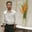Related Tags

c
c++
functions
community creator

# What are functors in C++?Behzad Ahmad

### Overview

In C++, a functor(function object) is the object of a class or struct, which we can call a function.

It overloads the function-call operator() so that it allows us to use the object as a function.

### Create a functor in C++

class Test
{
public:
void operator()()
{
// function body
}
};
int main()
{
Test temp;
temp();
}


Here in this syntax, we’re overloading the function call operator (). This would help us to call the object just like the function.

### Example

#include <iostream>
using namespace std;
class Test
{
public:
void operator()()
{
cout << "Basic Example of C++ Functors";
}
};
int main()
{
Test temp;
temp();
return 0;
}
Creating a functor in C++

### Explanation

• Line –2: We import the standard input and output libraries.

• Line 3: We create a Test class.

• Line 6–9: We overload the function call operator (), which simply prints a message.

• Line 11: We write a main driver for the program.

• Line 13: We create an object of the Test class, which is known as a temp.

• Line 14: We call the temp object by using the () operator.

RELATED TAGS

c
c++
functions
community creator

CONTRIBUTORBehzad Ahmad
RELATED COURSES

View all Courses

Keep Exploring

Learn in-demand tech skills in half the time